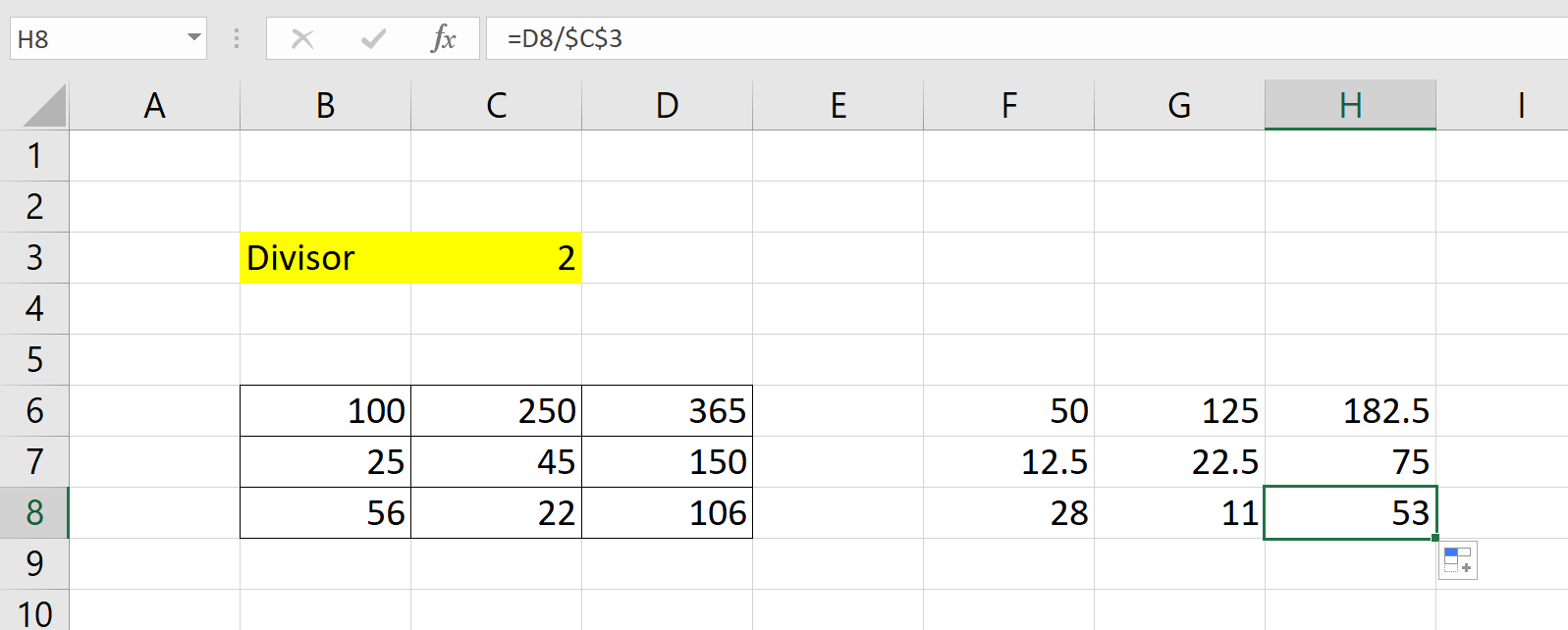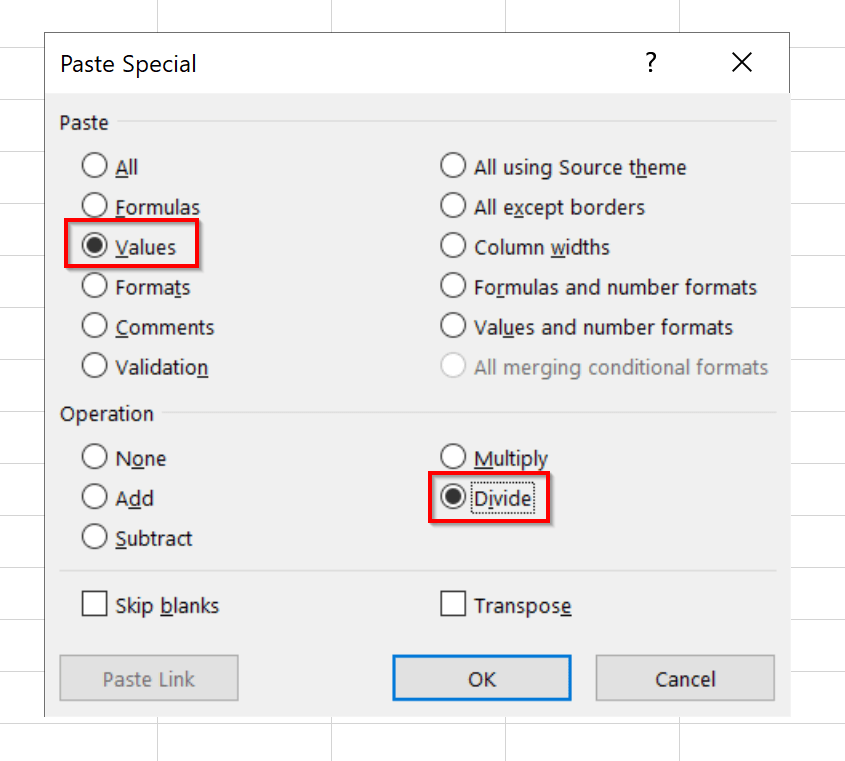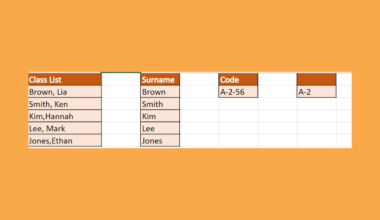# How to Divide a Range of Cells by a Number in Excel

This guide will show you how you can divide a range of cells by a number in Excel.

This operation is useful if you need to scale down an entire range of values. We will show you how to do this using the Paste Special tool and your typical Excel formulas.

Let’s take a look at a quick example where we might need to divide a range of cells by a specific number.

We have a list of values that range from 1 to 200. These values correspond to examination results for a test given to college students. We want to divide all values by 200 to get the percentage.

How do we do this easily in Microsoft Excel?

There are two quick methods to get the result we need. First, we can use the Paste Special option to apply a Divide operation on the entire range. Second, we can use a formula to create a second range of quotients from the input range.

To better understand how useful this operation is, let’s look at some examples of this formula working in Excel.

## A Real Example of Dividing a Range of Cells by a Number in Excel

This section will show a real example of dividing a range of cells in an Excel spreadsheet.

In the example below, we have a range of values in B6:D8. Using simple Excel formulas, we can create another range of values, but all the numbers have been halved this time.To get the values in Column C, we just need to use the following formula:

`=B6/\$C\$3`

The formula above will give us the upper-leftmost value in the range. We created an absolute reference to cell C3 so that we can fill the rest of the range without changing the divisor.In the example below, we’ve used the same technique to divide the range B12:C17 by 200. The result is equivalent to the percentage of the original value with respect to the maximum score of 200.We can change the formatting of the new range to create a more readable percentage.All the sheets above will also work with the Paste Special tool. If you’re ready to learn about how you can achieve this in Excel, head over to the next section!

## How to Divide a Range of Cells by a Number in Excel

This section will explain each step needed to divide an entire range of cells by a single number. You’ll learn how we can use the Paste Special tool and basic Excel formulas to achieve this result.

First, we’ll explain how you can use the Paste Special technique.

1. Select the cell that holds the divisor. This is the number we want to divide the range by. In this example, we’ll be dividing the range B6:D8 by two.2. After selecting the cell, press Ctrl + C to copy the cell’s contents.3. Next, select the entire range we’ll be dividing by two. In the example below, we’ve selected the cell range B6:D8.4. Right-click anywhere within the range to bring up the menu. Select the Paste Special… option. We can also press Ctrl + Alt + V to bring up the Paste Special dialog menu.5. In the Past Special pop-up menu, select the following settings below. We will select Values to indicate that we want the numerical value we copied earlier for the Paste option. We select Divide for the Operation option. This allows values within the range to be divided by the number we’ll be “pasting” in this action.Click
OK to perform the operation.
6. Your original range should now be divided by the specified number. In the example below, you can see that the range has been halved.Alternatively, we can use Excel formulas to create a new range. This has the benefit of keeping the original values. We can also dynamically change the divisor later on if needed.

1. We simply have to divide each cell by the given divisor using cell references for this to work. To easily fill out the range later on, we can make an absolute reference to our divisor so that it remains the same value.2. In the example below, we expanded the formula outward to achieve the same result.3. Changing the value of our divisor will dynamically change the values in our new range as well.1. What is the difference between Paste and Paste Special?
The Paste Special feature is more powerful than your typical Paste operation. This feature gives you control over whether to copy the value, formatting or other source cell properties when pasting to another cell. The Paste Special feature also lets you perform operations on target cells, which is why it’s useful for dividing a range of cells.

That’s all you need to remember to start using the Paste Special tool in Microsoft Excel to divide an entire range. This step-by-step guide shows how easy it is to scale multiple values down by a certain number. We can use this tip to find percentages easily.

Using functions to divide a range of cells by a single number is just one example of a time-saving trick that you can apply in Excel. With so many other Excel functions out there, you can surely find a few that makes your spreadsheets more powerful.

Are you interested in learning more about what Excel can do? Make sure to subscribe to our newsletter to be the first to know about the latest guides and tutorials from us.### Get emails from us about Microsoft Excel.

Our goal this year is to create lots of rich, bite-sized tutorials for Microsoft Excel users like you. If you liked this one, you'd love what we are working on! Readers receive ✨ early access ✨ to new content.

##### You May Also Like## How to Convert Decimals to Fractions in Excel

This guide will explain how you can convert decimals to fractions in Excel. Excel includes functions and custom…## How to Calculate Conditional Probability in Excel

This is the ultimate guide on how to calculate conditional probability in Excel using two easy and efficient…## How to Use TEXTBEFORE Function in Excel

This guide will discuss how to use the TEXTBEFORE function in Excel.  The rules for using the TEXTBEFORE…## How to Create a Population Pyramid Chart in Excel

A population pyramid chart is useful when you indeed to illustrate the distribution of a population by age…